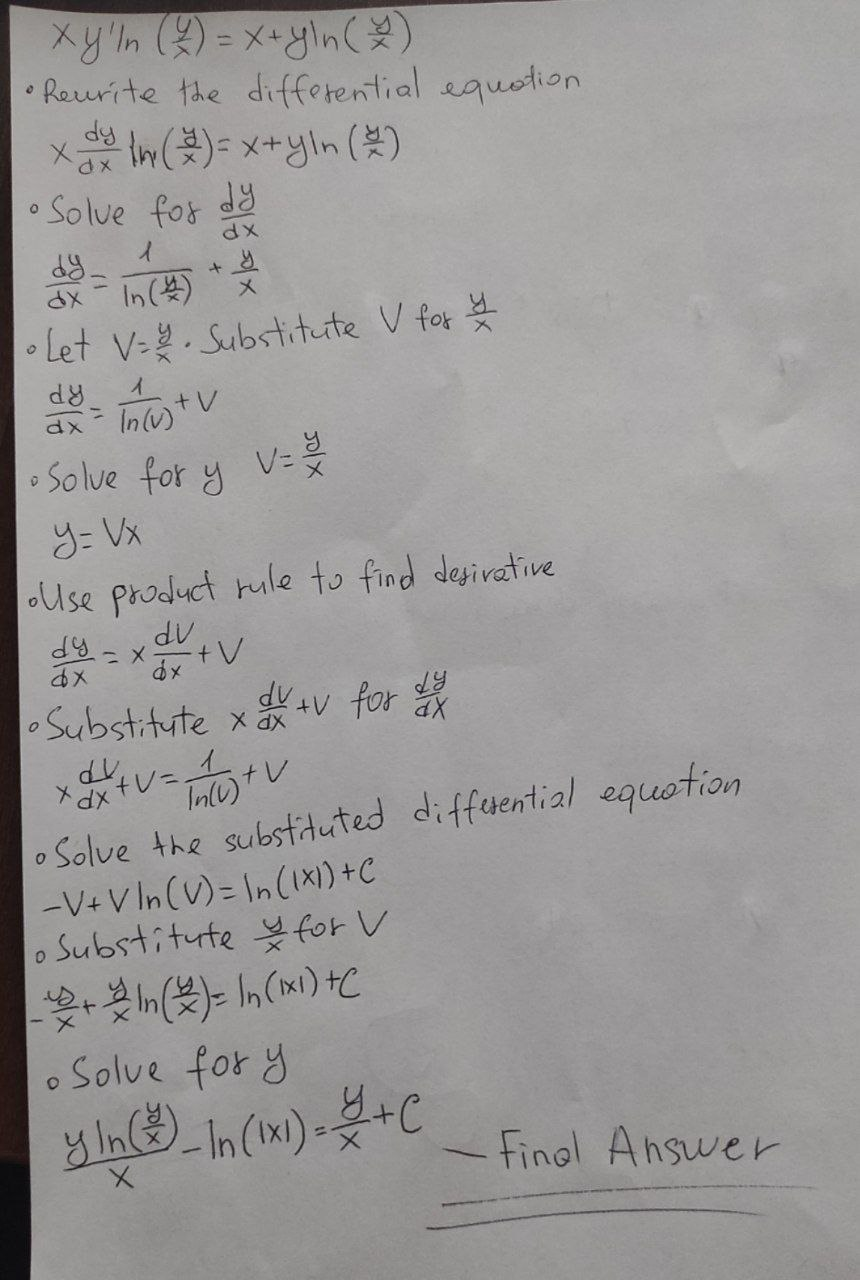# xy&#39;ln(y/x)=x+yln(y/x) find general solution of the homogenous equation &nbsp;

xy'ln(y/x)=x+yln(y/x)find general solution of the homogenous equation
You can still ask an expert for help

## Want to know more about Discrete math?

• Questions are typically answered in as fast as 30 minutes

Solve your problem for the price of one coffee

• Math expert for every subject
• Pay only if we can solve itVasquez